﻿ Meet our 2019 Finalists and Judges

 Like Tweet
 /* styles */ August 28, 2019 Dear Readers, It is that exciting time of year for our readers when the Dayton Literary Peace Prize finalists are announced. Our 78 first readers have winnowed the nominations down to six books in each category and have determined which of the very fine nominations best fit our dual criteria of high literary quality and a strong connection to the concept of peace. Dayton needs these books right now. The world needs these books right now. As Dayton is in the process of healing from the violence of nature and the tragedy of the shootings in the Oregon District, these books help with the healing. As a group, they show us that nationally and internationally, historically and currently, violence has ravaged lives, families, and countries—but in each book there is hope for finding peace and reconciliation. Often that hope comes from the strength of personal connections—connections with others who are sharing the struggle for peace. Dayton Strong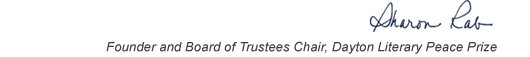table div table+table+table+table div table{width:100%;padding:0}table div table+table+table+table div table img{width:96.23%;padding:0;float:none}table div table+table+table+table div table td{width:100%;padding:0 1.88% 18px}/* styles */# Our Finalists - Fiction

 table div table+table+table+table+table+table div table{width:100%;padding:0}table div table+table+table+table+table+table div table img{width:96.23%;padding:0;float:none}table div table+table+table+table+table+table div table td{width:100%;padding:0 1.88% 18px}/* styles */Insurrecto by Gina Apostol, Soho Press: Histories and personalities collide in this literary tour-de-force about the Philippines’ present and America’s past. Two women, a Filipino translator and an American filmmaker, go on a road trip in Duterte’s Philippines, collaborating and clashing in the writing of a film script about a massacre during the Philippine-American War. Insurrecto contains within its dramatic action two rival scripts—one about a white photographer, the other about a Filipino school teacher.

 table div table+table+table+table+table+table+table+table div table{width:100%;padding:0}table div table+table+table+table+table+table+table+table div table img{width:96.23%;padding:0;float:none}table div table+table+table+table+table+table+table+table div table td{width:100%;padding:0 1.88% 18px}/* styles */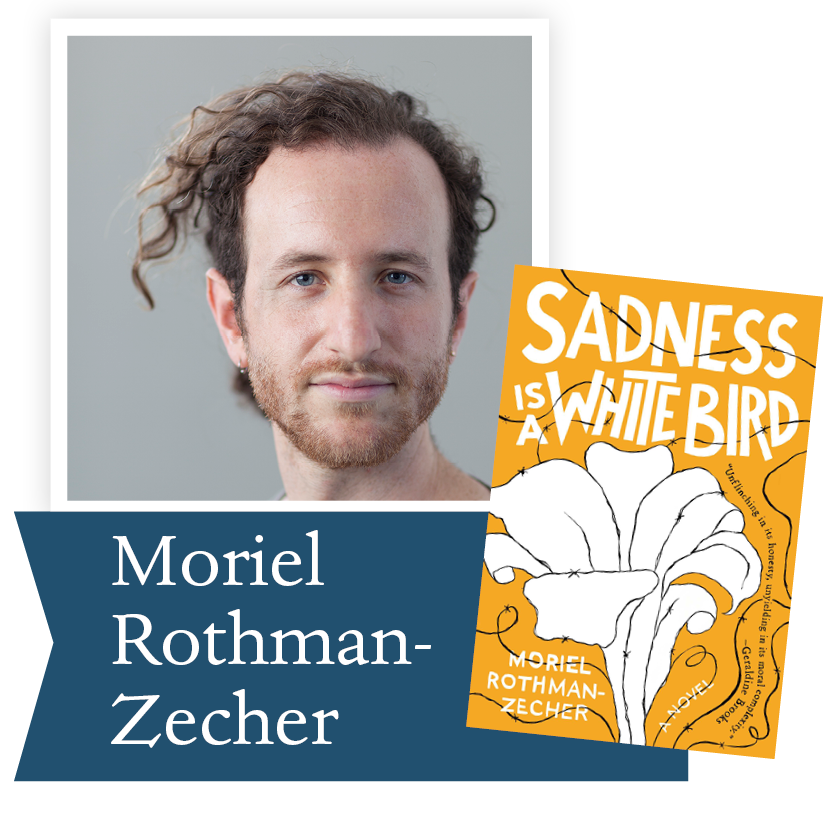Sadness Is A White Bird by Moriel Rothman-Zecher, Atria Books (S&S): In this debut novel from the MacDowell Colony fellow and National Book Foundation “5 Under 35” honoree, a young man prepares to serve in the Israeli army while also trying to reconcile his close relationship to two Palestinian siblings with his deeply ingrained loyalties to family and country. Powerful, important, and timely, Sadness Is A White Bird explores one man’s attempts to find a place for himself, discovering in the process a beautiful, against-the-odds love in the darkness of a never-ending conflict.

 table div table+table+table+table+table+table+table+table+table+table div table{width:100%;padding:0}table div table+table+table+table+table+table+table+table+table+table div table img{width:96.23%;padding:0;float:none}table div table+table+table+table+table+table+table+table+table+table div table td{width:100%;padding:0 1.88% 18px}/* styles */The Overstory by Richard Powers, WW Norton & Co: Winner of the 2019 Pulitzer Prize in Fiction, The Overstory is a sweeping, impassioned work of activism and resistance that is also a stunning evocation of—and paean to—the natural world. There is a world alongside ours—vast, slow, interconnected, resourceful, magnificently inventive, and almost invisible to us. This is the story of a handful of people who learn how to see that world and are drawn into its unfolding catastrophe.

 table div table+table+table+table+table+table+table+table+table+table+table+table div table{width:100%;padding:0}table div table+table+table+table+table+table+table+table+table+table+table+table div table img{width:96.23%;padding:0;float:none}table div table+table+table+table+table+table+table+table+table+table+table+table div table td{width:100%;padding:0 1.88% 18px}/* styles */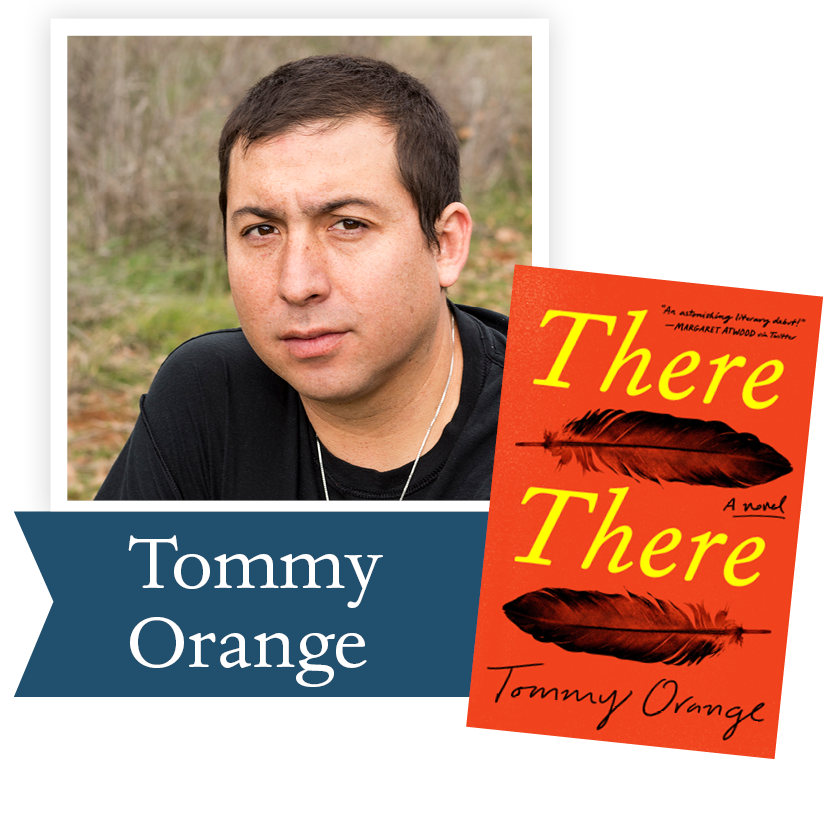There There by Tommy Orange, Knopf: Fierce, funny, suspenseful, and thoroughly modern, There There offers a kaleidoscopic look at Native American life in Oakland, California. Writing in a voice full of poetry and rage, exploding onto the page with urgency and force, Tommy Orange has created a stunning novel that grapples with a complex and painful history, with an inheritance of beauty and profound spirituality, and with a plague of addiction, abuse, and suicide.

 table div table+table+table+table+table+table+table+table+table+table+table+table+table+table div table{width:100%;padding:0}table div table+table+table+table+table+table+table+table+table+table+table+table+table+table div table img{width:96.23%;padding:0;float:none}table div table+table+table+table+table+table+table+table+table+table+table+table+table+table div table td{width:100%;padding:0 1.88% 18px}/* styles */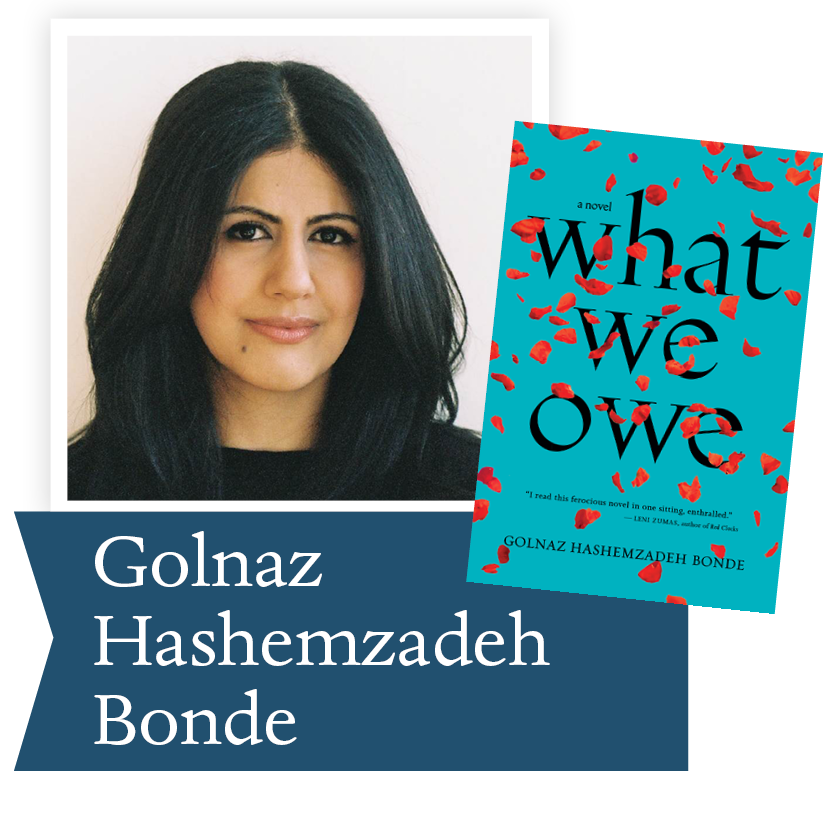What We Owe by Golnaz Hashemzadeh Bonde, Houghton, Mifflin, Harcourt: Here is an extraordinary story of exile, dislocation, and the emotional minefields between mothers and daughters; a story of love, guilt and dreams for a better future, vibrating with both sorrow and an unquenchable joie de vivre. With its startling honesty, dark wit, and irresistible momentum, What We Owe introduces a fierce and necessary new voice in international fiction.

 table div table+table+table+table+table+table+table+table+table+table+table+table+table+table+table+table div table{width:100%;padding:0}table div table+table+table+table+table+table+table+table+table+table+table+table+table+table+table+table div table img{width:96.23%;padding:0;float:none}table div table+table+table+table+table+table+table+table+table+table+table+table+table+table+table+table div table td{width:100%;padding:0 1.88% 18px}/* styles */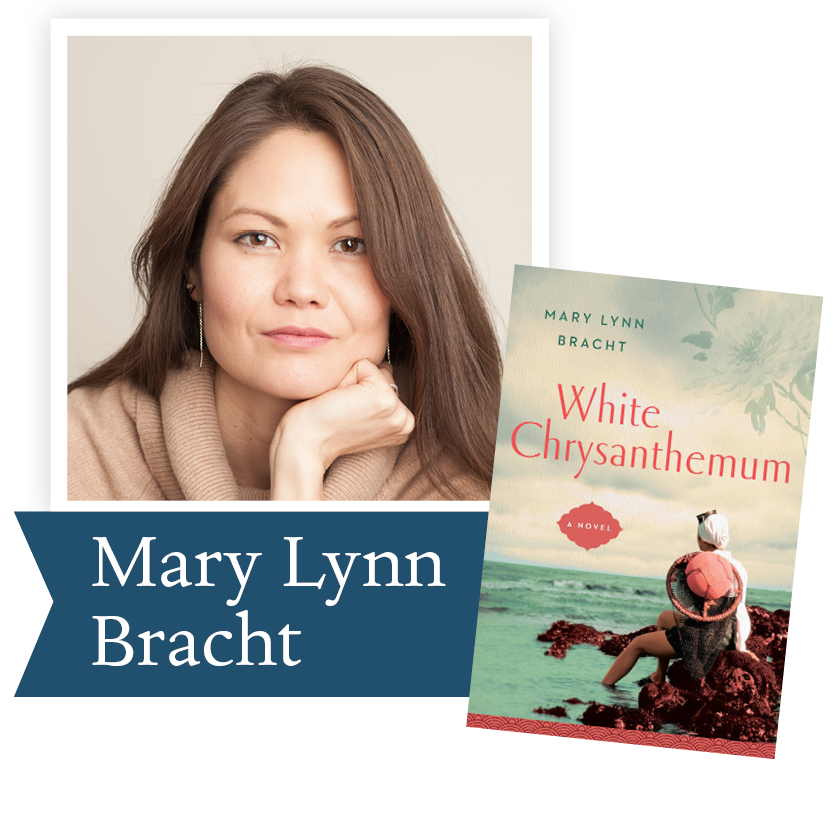White Chrysanthemum by Mary Lynn Bracht, GP Putnam’s Sons, PRH: White Chrysanthemum brings to life the heartbreaking history of Korea through the deeply moving and redemptive story of two sisters separated by World War II. It is a moving fictional account of a shockingly pervasive real-life assault—the sexual slavery of an estimated 200,000 Korean women during the Second World War.

 table div table+table+table+table+table+table+table+table+table+table+table+table+table+table+table+table+table+table div table{width:100%;padding:0}table div table+table+table+table+table+table+table+table+table+table+table+table+table+table+table+table+table+table div table img{width:96.23%;padding:0;float:none}table div table+table+table+table+table+table+table+table+table+table+table+table+table+table+table+table+table+table div table td{width:100%;padding:0 1.88% 18px}/* styles */# Our Finalists - Nonfiction

 table div table+table+table+table+table+table+table+table+table+table+table+table+table+table+table+table+table+table+table+table div table{width:100%;padding:0}table div table+table+table+table+table+table+table+table+table+table+table+table+table+table+table+table+table+table+table+table div table img{width:96.23%;padding:0;float:none}table div table+table+table+table+table+table+table+table+table+table+table+table+table+table+table+table+table+table+table+table div table td{width:100%;padding:0 1.88% 18px}/* styles */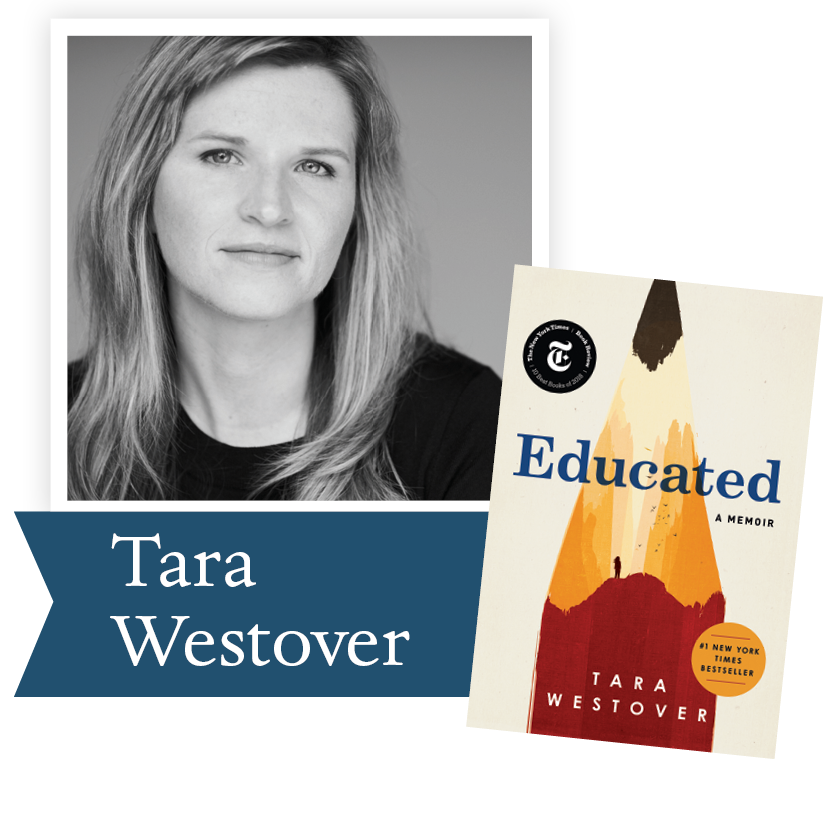Educated by Tara Westover, Random House: With the acute insight that distinguishes all great writers, Westover has crafted a universal coming-of-age story that gets to the heart of what an education offers: the perspective to see one's life through new eyes, and the will to change it.

 table div table+table+table+table+table+table+table+table+table+table+table+table+table+table+table+table+table+table+table+table+table+table div table{width:100%;padding:0}table div table+table+table+table+table+table+table+table+table+table+table+table+table+table+table+table+table+table+table+table+table+table div table img{width:96.23%;padding:0;float:none}table div table+table+table+table+table+table+table+table+table+table+table+table+table+table+table+table+table+table+table+table+table+table div table td{width:100%;padding:0 1.88% 18px}/* styles */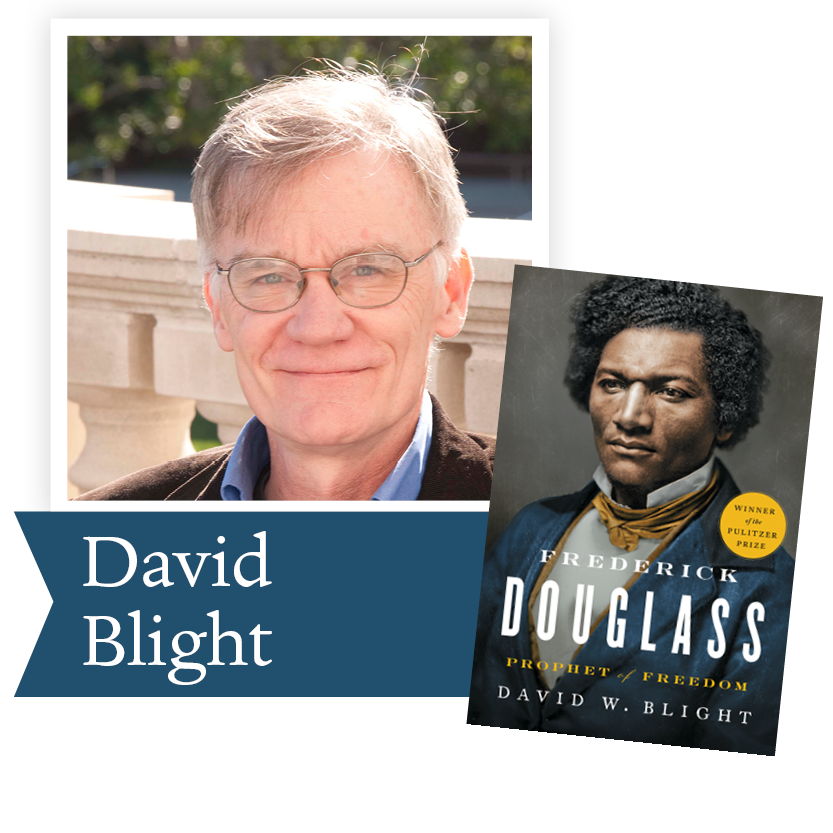Frederick Douglass by David Blight, Simon and Schuster: In his “cinematic and deeply engaging” (The New York Times Book Review) biography, Blight has drawn on new information held in a private collection that few other historians have consulted, as well as recently discovered issues of Douglass’s newspapers. Blight’s Pulitzer Prize-winning book tells the fascinating story of Douglass’s two marriages and his complex extended family.

 table div table+table+table+table+table+table+table+table+table+table+table+table+table+table+table+table+table+table+table+table+table+table+table+table div table{width:100%;padding:0}table div table+table+table+table+table+table+table+table+table+table+table+table+table+table+table+table+table+table+table+table+table+table+table+table div table img{width:96.23%;padding:0;float:none}table div table+table+table+table+table+table+table+table+table+table+table+table+table+table+table+table+table+table+table+table+table+table+table+table div table td{width:100%;padding:0 1.88% 18px}/* styles */I Should Have Honor by Khalida Brohi, Random House: A fearless memoir about tribal life in Pakistan—and the act of violence that inspired one ambitious young woman to pursue a life of activism and female empowerment. And ultimately, she learned that the only way to eradicate the parts of a culture she despised was to fully embrace the parts of it that she loved.

 table div table+table+table+table+table+table+table+table+table+table+table+table+table+table+table+table+table+table+table+table+table+table+table+table+table+table div table{width:100%;padding:0}table div table+table+table+table+table+table+table+table+table+table+table+table+table+table+table+table+table+table+table+table+table+table+table+table+table+table div table img{width:96.23%;padding:0;float:none}table div table+table+table+table+table+table+table+table+table+table+table+table+table+table+table+table+table+table+table+table+table+table+table+table+table+table div table td{width:100%;padding:0 1.88% 18px}/* styles */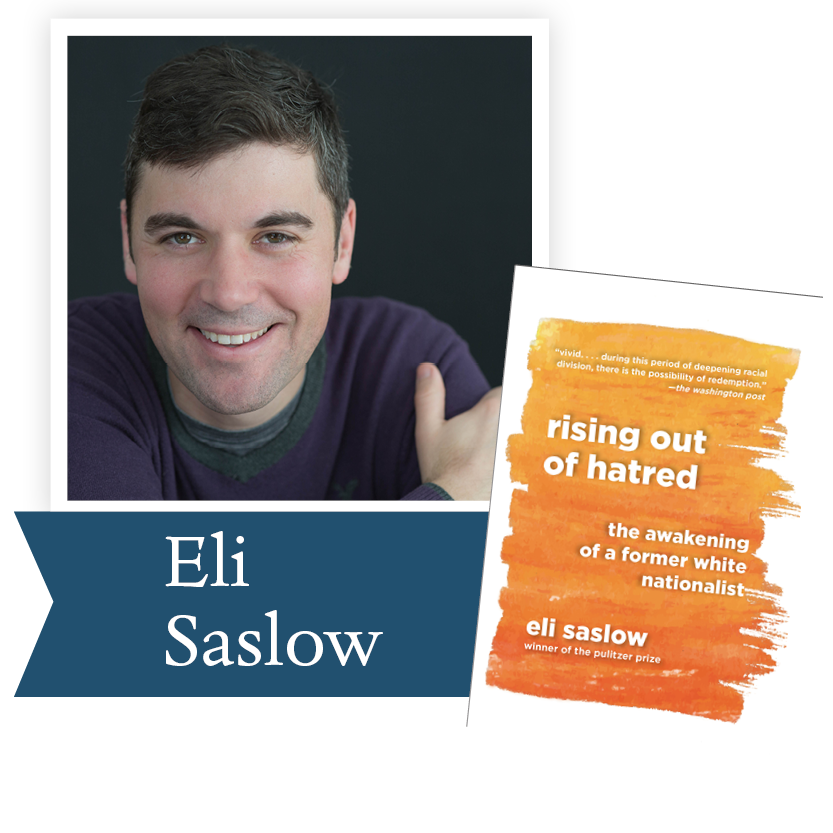Rising Out of Hatred by Eli Saslow, Doubleday: From a Pulitzer Prize-winning reporter, the powerful story of how a prominent white supremacist changed his heart and mind. With great empathy and narrative verve, Eli Saslow asks what Derek's story can tell us about America's increasingly divided nature. This is a book to help us understand the American moment and to help us better understand one another.

 table div table+table+table+table+table+table+table+table+table+table+table+table+table+table+table+table+table+table+table+table+table+table+table+table+table+table+table+table div table{width:100%;padding:0}table div table+table+table+table+table+table+table+table+table+table+table+table+table+table+table+table+table+table+table+table+table+table+table+table+table+table+table+table div table img{width:96.23%;padding:0;float:none}table div table+table+table+table+table+table+table+table+table+table+table+table+table+table+table+table+table+table+table+table+table+table+table+table+table+table+table+table div table td{width:100%;padding:0 1.88% 18px}/* styles */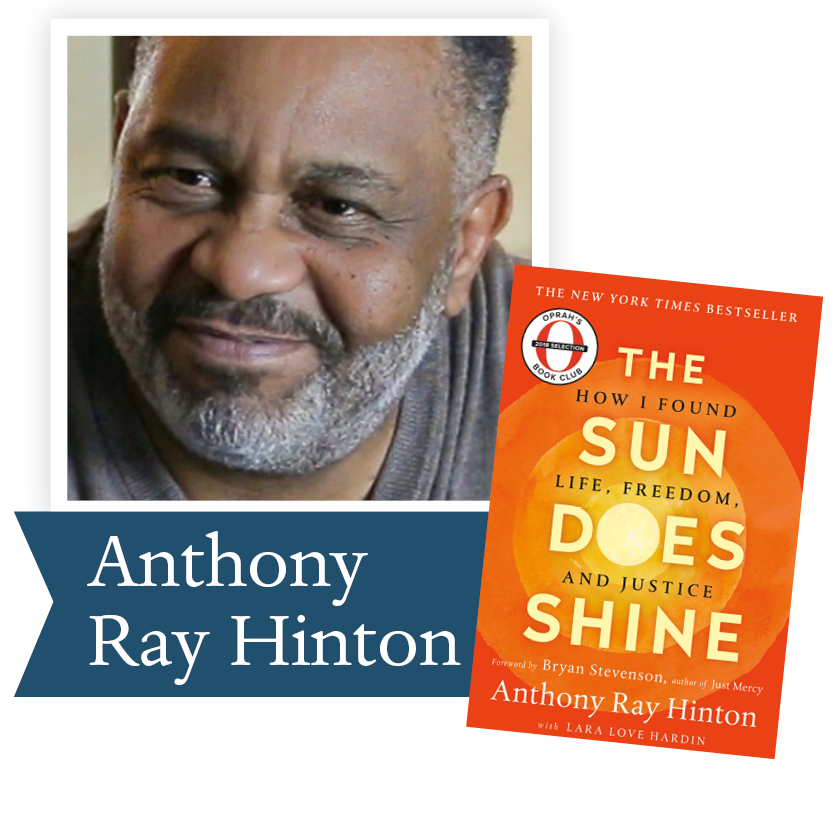● The Sun Does Shine: How I Found Life and Freedom on Death Row by Anthony Ray Hinton with Laura Love Hardin, St. Martins: With a foreword by Bryan Stevenson, The Sun Does Shine is an extraordinary testament to the power of hope sustained through the darkest times. Destined to be a classic memoir of wrongful imprisonment and freedom won, The Sun Does Shine tells Hinton’s dramatic 30-year journey and shows how you can take away a man’s freedom, but you can’t take away his imagination, humor, or joy.

 table div table+table+table+table+table+table+table+table+table+table+table+table+table+table+table+table+table+table+table+table+table+table+table+table+table+table+table+table+table+table div table{width:100%;padding:0}table div table+table+table+table+table+table+table+table+table+table+table+table+table+table+table+table+table+table+table+table+table+table+table+table+table+table+table+table+table+table div table img{width:96.23%;padding:0;float:none}table div table+table+table+table+table+table+table+table+table+table+table+table+table+table+table+table+table+table+table+table+table+table+table+table+table+table+table+table+table+table div table td{width:100%;padding:0 1.88% 18px}/* styles */Tigerland by Wil Haygood, Knopf: From the author of the best-selling The Butler–an emotional, inspiring story of two teams from a poor, black, segregated high school in Ohio, who, in the midst of the racial turbulence of 1968/1969, win the Ohio state baseball and basketball championships in the same year.

 table div table+table+table+table+table+table+table+table+table+table+table+table+table+table+table+table+table+table+table+table+table+table+table+table+table+table+table+table+table+table+table+table div table{width:100%;padding:0}table div table+table+table+table+table+table+table+table+table+table+table+table+table+table+table+table+table+table+table+table+table+table+table+table+table+table+table+table+table+table+table+table div table img{width:96.23%;padding:0;float:none}table div table+table+table+table+table+table+table+table+table+table+table+table+table+table+table+table+table+table+table+table+table+table+table+table+table+table+table+table+table+table+table+table div table td{width:100%;padding:0 1.88% 18px}/* styles */## Read the full press release here.

 table div table+table+table+table+table+table+table+table+table+table+table+table+table+table+table+table+table+table+table+table+table+table+table+table+table+table+table+table+table+table+table+table+table+table div table{width:100%;padding:0}table div table+table+table+table+table+table+table+table+table+table+table+table+table+table+table+table+table+table+table+table+table+table+table+table+table+table+table+table+table+table+table+table+table+table div table img{width:96.23%;padding:0;float:none}table div table+table+table+table+table+table+table+table+table+table+table+table+table+table+table+table+table+table+table+table+table+table+table+table+table+table+table+table+table+table+table+table+table+table div table td{width:100%;padding:0 1.88% 18px}/* styles */# Meet our Judges

 table div table+table+table+table+table+table+table+table+table+table+table+table+table+table+table+table+table+table+table+table+table+table+table+table+table+table+table+table+table+table+table+table+table+table+table+table div table{width:100%;padding:0}table div table+table+table+table+table+table+table+table+table+table+table+table+table+table+table+table+table+table+table+table+table+table+table+table+table+table+table+table+table+table+table+table+table+table+table+table div table img{width:96.23%;padding:0;float:none}table div table+table+table+table+table+table+table+table+table+table+table+table+table+table+table+table+table+table+table+table+table+table+table+table+table+table+table+table+table+table+table+table+table+table+table+table div table td{width:100%;padding:0 1.88% 18px}/* styles */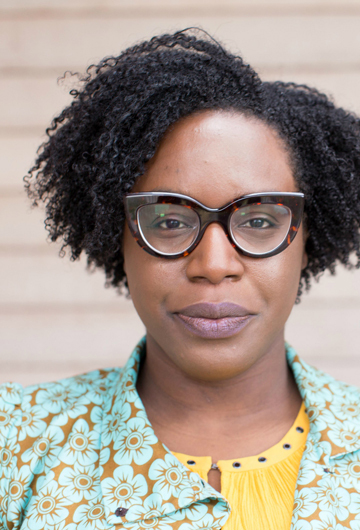Lesley Arimah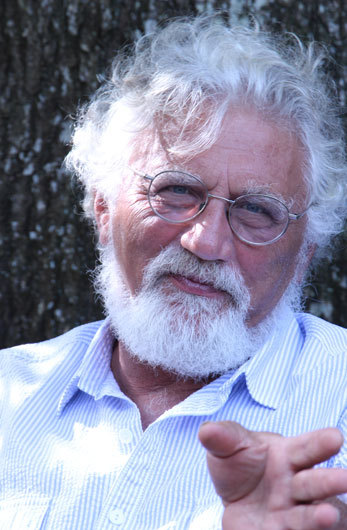Bob Shacochis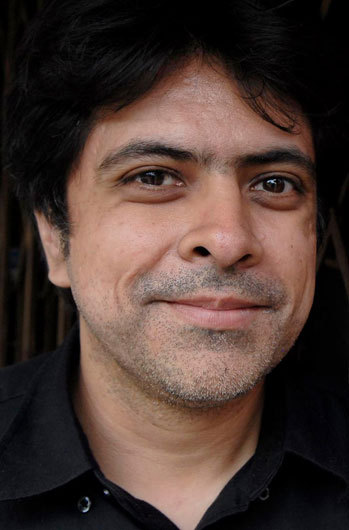Skyhorse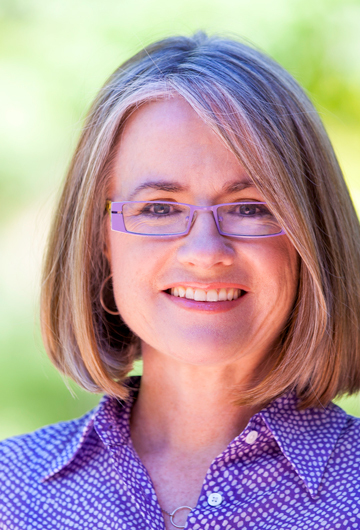Helen Thorpe
 table div table+table+table+table+table+table+table+table+table+table+table+table+table+table+table+table+table+table+table+table+table+table+table+table+table+table+table+table+table+table+table+table+table+table+table+table+table+table div table{width:100%;padding:0}table div table+table+table+table+table+table+table+table+table+table+table+table+table+table+table+table+table+table+table+table+table+table+table+table+table+table+table+table+table+table+table+table+table+table+table+table+table+table div table img{width:96.23%;padding:0;float:none}table div table+table+table+table+table+table+table+table+table+table+table+table+table+table+table+table+table+table+table+table+table+table+table+table+table+table+table+table+table+table+table+table+table+table+table+table+table+table div table td{width:100%;padding:0 1.88% 18px}/* styles */## Fiction Judges

 table div table+table+table+table+table+table+table+table+table+table+table+table+table+table+table+table+table+table+table+table+table+table+table+table+table+table+table+table+table+table+table+table+table+table+table+table+table+table+table+table div table{width:100%;padding:0}table div table+table+table+table+table+table+table+table+table+table+table+table+table+table+table+table+table+table+table+table+table+table+table+table+table+table+table+table+table+table+table+table+table+table+table+table+table+table+table+table div table img{width:96.23%;padding:0;float:none}table div table+table+table+table+table+table+table+table+table+table+table+table+table+table+table+table+table+table+table+table+table+table+table+table+table+table+table+table+table+table+table+table+table+table+table+table+table+table+table+table div table td{width:100%;padding:0 1.88% 18px}/* styles *//* styles */ Lesley Nneka Arimah was born in the UK and grew up in Nigeria and wherever else her father was stationed for work. She has been a finalist for a National Magazine Award and the Caine Prize, and a winner of the African Commonwealth Short Story Prize, an O. Henry Award, and other honors. Her work has appeared in The New Yorker, Harper’s, GRANTA and has received support from The Elizabeth George Foundation, The Jerome Foundation, and MacDowell, among others. She was selected for the National Book Foundation’s 5 Under 35 and her debut collection WHAT IT MEANS WHEN A MAN FALLS FROM THE SKY won the 2017 Kirkus Prize. She lives in Minneapolis and is working on a novel about you.
 table div table+table+table+table+table+table+table+table+table+table+table+table+table+table+table+table+table+table+table+table+table+table+table+table+table+table+table+table+table+table+table+table+table+table+table+table+table+table+table+table+table+table div table{width:100%;padding:0}table div table+table+table+table+table+table+table+table+table+table+table+table+table+table+table+table+table+table+table+table+table+table+table+table+table+table+table+table+table+table+table+table+table+table+table+table+table+table+table+table+table+table div table img{width:96.23%;padding:0;float:none}table div table+table+table+table+table+table+table+table+table+table+table+table+table+table+table+table+table+table+table+table+table+table+table+table+table+table+table+table+table+table+table+table+table+table+table+table+table+table+table+table+table+table div table td{width:100%;padding:0 1.88% 18px}/* styles *//* styles */ Bob Shacochis is the winner of the Dayton Literary Peace Prize 2014 Award in Fiction for The Woman Who Lost Her Soul. A Pulitzer Prize finalist, the book was selected as a Best Book of the Year by The Washington Post, NPR, Amazon.com, and others. His first collection of stories, Easy in the Islands, won the National Book Award for First Fiction, and his second collection, The Next New World, was awarded the Prix de Rome from the American Academy of Arts and Letters. Shacochis is also the author of the novel Swimming in the Volcano, a finalist for the National Book Award, and The Immaculate Invasion, a work of literary reportage that was a finalist for The New Yorker Book Award for Best Nonfiction of the Year. Read more...
 table div table+table+table+table+table+table+table+table+table+table+table+table+table+table+table+table+table+table+table+table+table+table+table+table+table+table+table+table+table+table+table+table+table+table+table+table+table+table+table+table+table+table+table+table div table{width:100%;padding:0}table div table+table+table+table+table+table+table+table+table+table+table+table+table+table+table+table+table+table+table+table+table+table+table+table+table+table+table+table+table+table+table+table+table+table+table+table+table+table+table+table+table+table+table+table div table img{width:96.23%;padding:0;float:none}table div table+table+table+table+table+table+table+table+table+table+table+table+table+table+table+table+table+table+table+table+table+table+table+table+table+table+table+table+table+table+table+table+table+table+table+table+table+table+table+table+table+table+table+table div table td{width:100%;padding:0 1.88% 18px}/* styles */## Nonfiction Judges

 table div table+table+table+table+table+table+table+table+table+table+table+table+table+table+table+table+table+table+table+table+table+table+table+table+table+table+table+table+table+table+table+table+table+table+table+table+table+table+table+table+table+table+table+table+table+table div table{width:100%;padding:0}table div table+table+table+table+table+table+table+table+table+table+table+table+table+table+table+table+table+table+table+table+table+table+table+table+table+table+table+table+table+table+table+table+table+table+table+table+table+table+table+table+table+table+table+table+table+table div table img{width:96.23%;padding:0;float:none}table div table+table+table+table+table+table+table+table+table+table+table+table+table+table+table+table+table+table+table+table+table+table+table+table+table+table+table+table+table+table+table+table+table+table+table+table+table+table+table+table+table+table+table+table+table+table div table td{width:100%;padding:0 1.88% 18px}/* styles *//* styles */ Skyhorse's debut novel, The Madonnas of Echo Park (Simon & Schuster, 2010), received the 2011 PEN/Hemingway Award, and the Sue Kaufman Prize for First Fiction from the American Academy of Arts and Letters. The book was also a Barnes & Noble Discover Great New Writers pick. Take This Man: A Memoir (Simon & Schuster, 2014) was an Amazon Best Book of the Month selection and named by Kirkus Reviews as one the Best Nonfiction Books of the year. Skyhorse has also co-edited an anthology, We Wear The Mask: 15 True Stories Of Passing in America (Beacon Press, 2017). He has been awarded fellowships at Ucross Foundation, the Breadloaf Writers’ Conference, and was the 2014-2015 Jenny McKean Moore Writer-In-Washington at George Washington University. Skyhorse is an Associate Professor of English at Indiana University in Bloomington.
 table div table+table+table+table+table+table+table+table+table+table+table+table+table+table+table+table+table+table+table+table+table+table+table+table+table+table+table+table+table+table+table+table+table+table+table+table+table+table+table+table+table+table+table+table+table+table+table+table div table{width:100%;padding:0}table div table+table+table+table+table+table+table+table+table+table+table+table+table+table+table+table+table+table+table+table+table+table+table+table+table+table+table+table+table+table+table+table+table+table+table+table+table+table+table+table+table+table+table+table+table+table+table+table div table img{width:96.23%;padding:0;float:none}table div table+table+table+table+table+table+table+table+table+table+table+table+table+table+table+table+table+table+table+table+table+table+table+table+table+table+table+table+table+table+table+table+table+table+table+table+table+table+table+table+table+table+table+table+table+table+table+table div table td{width:100%;padding:0 1.88% 18px}/* styles *//* styles */ Helen Thorpe was born in London to Irish parents. She was formerly a staff writer for The New York Observer, The New Yorker’s Talk of the Town section, and Texas Monthly. She is the author of three books, Just Like Us, Soldier Girls, and The Newcomers. Her books are works of narrative nonfiction that document in a human and intimate way the lives of immigrants, refugees, and veterans of foreign conflicts. Thorpe’s magazine journalism has appeared in The New York Times Magazine, The New Yorker, Texas Monthly, Westword, Chalkbeat, and 5280. She lives in Denver, Colorado.
 table div table+table+table+table+table+table+table+table+table+table+table+table+table+table+table+table+table+table+table+table+table+table+table+table+table+table+table+table+table+table+table+table+table+table+table+table+table+table+table+table+table+table+table+table+table+table+table+table+table+table div table{width:100%;padding:0}table div table+table+table+table+table+table+table+table+table+table+table+table+table+table+table+table+table+table+table+table+table+table+table+table+table+table+table+table+table+table+table+table+table+table+table+table+table+table+table+table+table+table+table+table+table+table+table+table+table+table div table img{width:96.23%;padding:0;float:none}table div table+table+table+table+table+table+table+table+table+table+table+table+table+table+table+table+table+table+table+table+table+table+table+table+table+table+table+table+table+table+table+table+table+table+table+table+table+table+table+table+table+table+table+table+table+table+table+table+table+table div table td{width:100%;padding:0 1.88% 18px}/* styles */## The DLPP’s 14th annual gala event is Sunday, November 3, and is sold out. There are still opportunities to meet our authors and hear them share insights about their books.

Sunday, November 3, 2019 ~ A Conversation with the Authors

9/16, 9/30 & 10/7, 2019 ~Dayton Metro Library

 table div table+table+table+table+table+table+table+table+table+table+table+table+table+table+table+table+table+table+table+table+table+table+table+table+table+table+table+table+table+table+table+table+table+table+table+table+table+table+table+table+table+table+table+table+table+table+table+table+table+table+table+table div table{width:100%;padding:0}table div table+table+table+table+table+table+table+table+table+table+table+table+table+table+table+table+table+table+table+table+table+table+table+table+table+table+table+table+table+table+table+table+table+table+table+table+table+table+table+table+table+table+table+table+table+table+table+table+table+table+table+table div table img{width:96.23%;padding:0;float:none}table div table+table+table+table+table+table+table+table+table+table+table+table+table+table+table+table+table+table+table+table+table+table+table+table+table+table+table+table+table+table+table+table+table+table+table+table+table+table+table+table+table+table+table+table+table+table+table+table+table+table+table+table div table td{width:100%;padding:0 1.88% 18px}/* styles */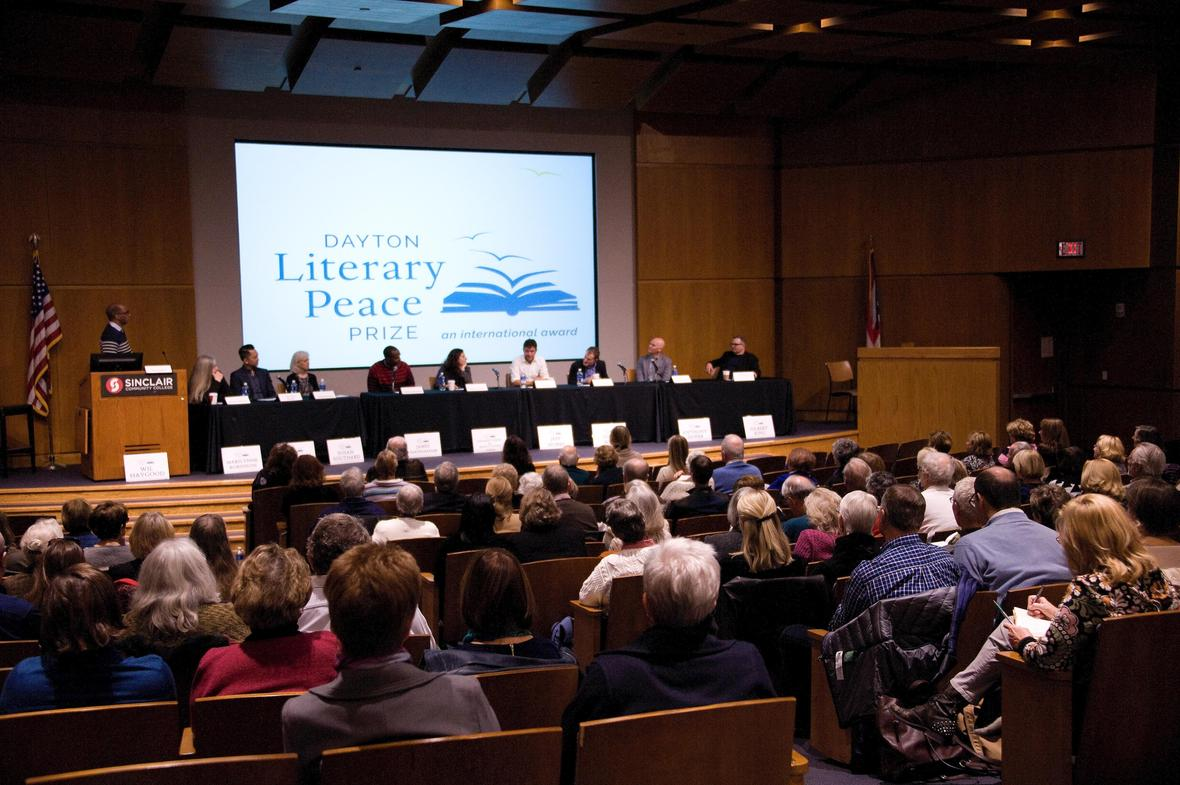## A Conversation with the 2019 Dayton Literary Peace Prize Winners

Limited seating: reservations accepted for the first 300. Register here.

Sunday, November 3, 2019
9:00 AM Registration; 10:00 AM Program

Sinclair Community College, Ponitz Center, Building 12

Book signing after the program; Books will be available for purchase. Free underground parking is available under Building 12 off of W. 4th Street.

 table div table+table+table+table+table+table+table+table+table+table+table+table+table+table+table+table+table+table+table+table+table+table+table+table+table+table+table+table+table+table+table+table+table+table+table+table+table+table+table+table+table+table+table+table+table+table+table+table+table+table+table+table+table+table div table{width:100%;padding:0}table div table+table+table+table+table+table+table+table+table+table+table+table+table+table+table+table+table+table+table+table+table+table+table+table+table+table+table+table+table+table+table+table+table+table+table+table+table+table+table+table+table+table+table+table+table+table+table+table+table+table+table+table+table+table div table img{width:96.23%;padding:0;float:none}table div table+table+table+table+table+table+table+table+table+table+table+table+table+table+table+table+table+table+table+table+table+table+table+table+table+table+table+table+table+table+table+table+table+table+table+table+table+table+table+table+table+table+table+table+table+table+table+table+table+table+table+table+table+table div table td{width:100%;padding:0 1.88% 18px}/* styles */## Join us for a DLPP Community Conversation at the Dayton Metro Library

All public conversations are from 6:30 to 8:00 pm in the Eichelberger Forum of the Main branch of the Dayton Metro Library. The library has ample copies of the books for checkout.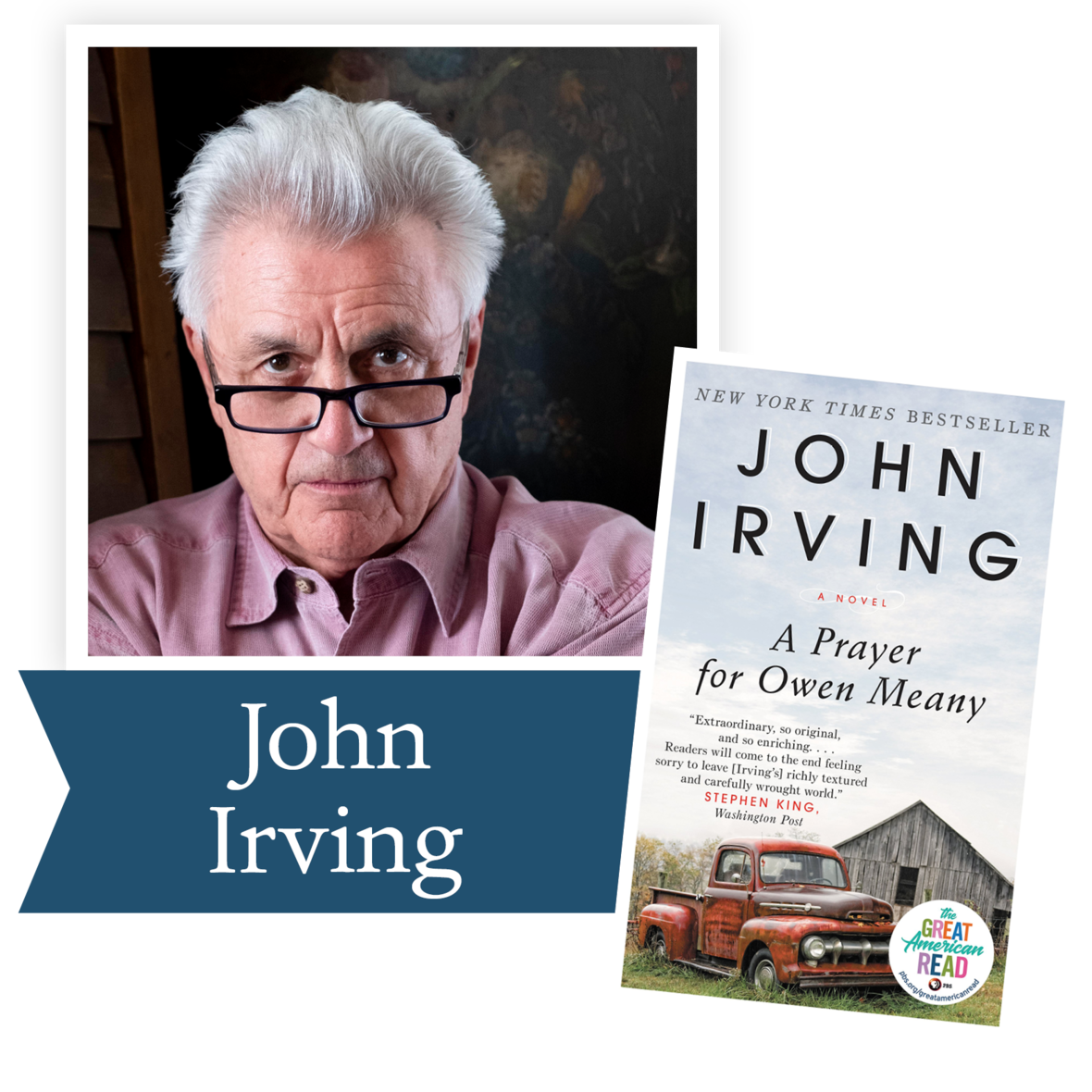9/16/2019
John Irving's A Prayer for Owen Meany

Public Intellectuals, Darren McGarvey of Kettering Fairmont High School and Carolyn Stoermer of Wright State University. The panel will include area educators and students. Discussion will include how to teach this work.

 table div table+table+table+table+table+table+table+table+table+table+table+table+table+table+table+table+table+table+table+table+table+table+table+table+table+table+table+table+table+table+table+table+table+table+table+table+table+table+table+table+table+table+table+table+table+table+table+table+table+table+table+table+table+table+table+table+table div table{width:100%;padding:0}table div table+table+table+table+table+table+table+table+table+table+table+table+table+table+table+table+table+table+table+table+table+table+table+table+table+table+table+table+table+table+table+table+table+table+table+table+table+table+table+table+table+table+table+table+table+table+table+table+table+table+table+table+table+table+table+table+table div table img{width:96.23%;padding:0;float:none}table div table+table+table+table+table+table+table+table+table+table+table+table+table+table+table+table+table+table+table+table+table+table+table+table+table+table+table+table+table+table+table+table+table+table+table+table+table+table+table+table+table+table+table+table+table+table+table+table+table+table+table+table+table+table+table+table+table div table td{width:100%;padding:0 1.88% 18px}/* styles */9/30/2019
Gloria Steinem's My Life on the Road

Public Intellectual, Emerita Professor Marybeth Pringle, of Wright State University. Panel will include an area public librarian and members of the Human Race Theatre involved in the spring production of Gloria: A Life. This is a timely book, given among other things that 2019 is the 100th anniversary of woman suffrage in the U.S.

 table div table+table+table+table+table+table+table+table+table+table+table+table+table+table+table+table+table+table+table+table+table+table+table+table+table+table+table+table+table+table+table+table+table+table+table+table+table+table+table+table+table+table+table+table+table+table+table+table+table+table+table+table+table+table+table+table+table+table+table div table{width:100%;padding:0}table div table+table+table+table+table+table+table+table+table+table+table+table+table+table+table+table+table+table+table+table+table+table+table+table+table+table+table+table+table+table+table+table+table+table+table+table+table+table+table+table+table+table+table+table+table+table+table+table+table+table+table+table+table+table+table+table+table+table+table div table img{width:96.23%;padding:0;float:none}table div table+table+table+table+table+table+table+table+table+table+table+table+table+table+table+table+table+table+table+table+table+table+table+table+table+table+table+table+table+table+table+table+table+table+table+table+table+table+table+table+table+table+table+table+table+table+table+table+table+table+table+table+table+table+table+table+table+table+table div table td{width:100%;padding:0 1.88% 18px}/* styles */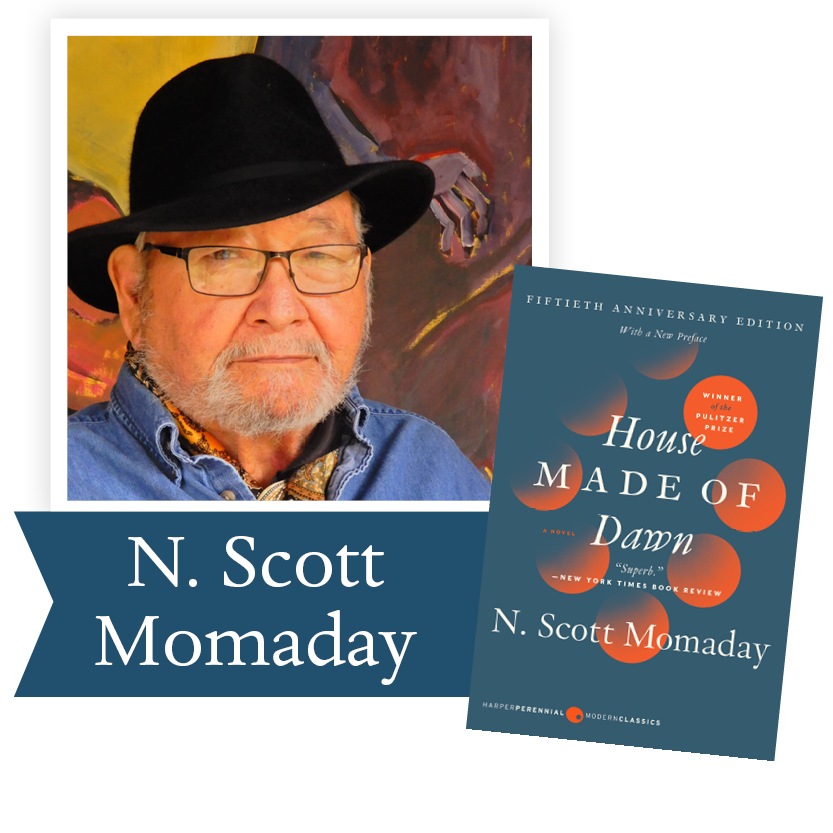10/7/2019